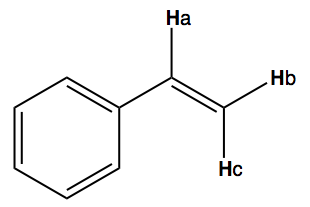# Problem: Predict the complex splitting patterns one would expect to find in the ethenyl substituent of the molecule below. Ha,b = 17HzHa,c = 11Hz Hb,c = 2Hz

###### FREE Expert Solution
90% (327 ratings)
###### Problem Details

Predict the complex splitting patterns one would expect to find in the ethenyl substituent of the molecule below.

Ha,b = 17Hz

Ha,c = 11Hz

Hb,c = 2HzFrequently Asked Questions

What scientific concept do you need to know in order to solve this problem?

Our tutors have indicated that to solve this problem you will need to apply the 1H NMR: Spin-Splitting Complex Tree Diagrams concept. You can view video lessons to learn 1H NMR: Spin-Splitting Complex Tree Diagrams. Or if you need more 1H NMR: Spin-Splitting Complex Tree Diagrams practice, you can also practice 1H NMR: Spin-Splitting Complex Tree Diagrams practice problems.

What is the difficulty of this problem?

Our tutors rated the difficulty ofPredict the complex splitting patterns one would expect to f...as high difficulty.

How long does this problem take to solve?

Our expert Organic tutor, Jonathan took 4 minutes and 9 seconds to solve this problem. You can follow their steps in the video explanation above.

What professor is this problem relevant for?

Based on our data, we think this problem is relevant for Professor Wolfe's class at UMICH.